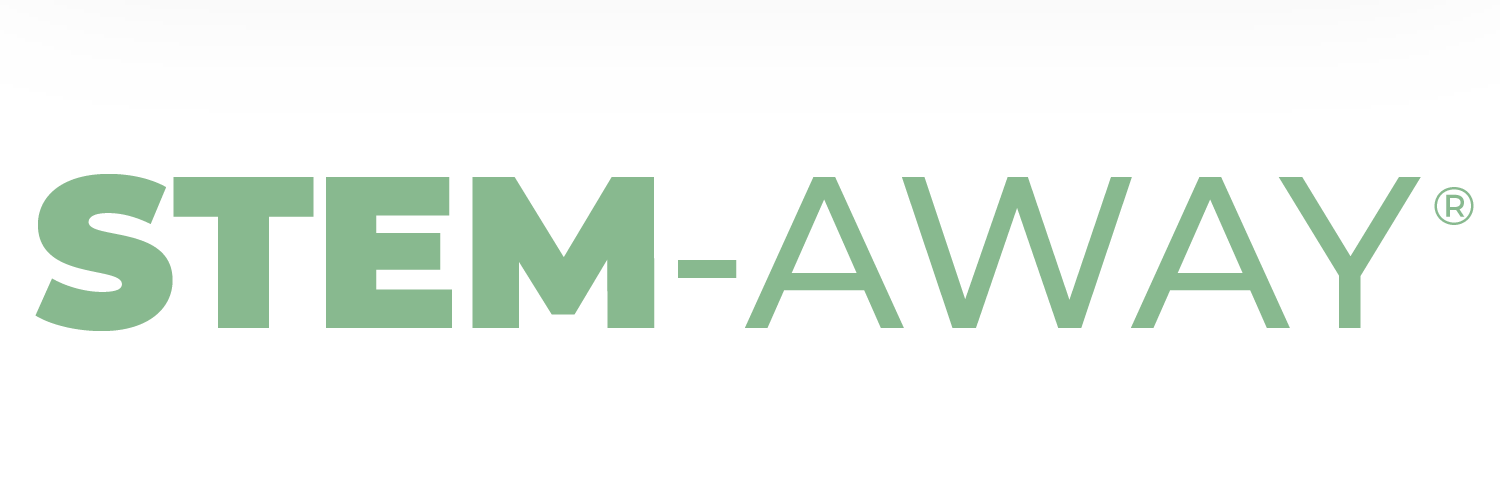# Machine Learning Level 1 - Module 4 - Dushyant Chaudhary

Overview of things learned:

1. Technical Area:
• Learned how to use SimpleTransformers library in the model
• Gained an understanding of combining an advanced model (BERT) and a simple one (logistic regression)
• Used matplotlib to plot some graphs about the data and learned about balancing data
1. Tools:
• Jupyter Notebooks
• SimpleTransformers
• Pandas, numpy, csv, matplotlib
1. Soft Skills: